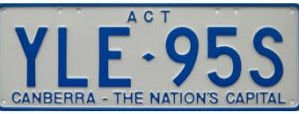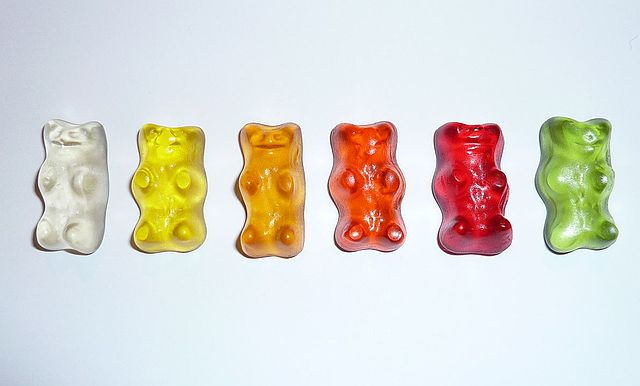# Arrangements

In many mathematics problems, we need to find the number of ways of arranging a set of objects in a straight line. Sometimes we are allowed to put the same object into the line two or more times, and other times we aren't.

In all cases, however, the order in which the objects appear in the line is important.

## Arrangements With Unlimited Repetition

The simplest arrangements to count are the ones in which the number of times each object occurs does not matter. There are no restrictions on which object can go in which position, and there are no restrictions on how many times each object appears.

To count these arrangements we use the following principle:

### The Fundamental Principle of Counting

If the first object can be placed in the first position in $a$ different ways, the second object can be placed in the second position in $b$ different ways, the third object can be placed in the third position in $c$ different ways, and so on, then the total number of arrangements of the objects is equal to the product $abc\dots$ of the numbers of ways of filling each position.

So, if we have $n$ objects that we wish to use to fill $r$ positions, and each object can be used as many different times as we like, then there are $n$ ways of filling each position, and the total number of arrangements of the objects is

$n \times n \times \dots \times n = n^r$

Let's try a few examples.

### Example 1

Suppose you need to come up with a new PIN (personal identification number) for your bank account. The PIN must have four digits. Any digit can be repeated as many times as you like. How many different PINs are possible?

Solution:

We need to find the number of arrangements of four digits out of $\{0,1,2,3,4,5,6,7,8,9\}$ in a line, where the order matters, and repetition is allowed.

Each of the four positions in the line can be filled in $10$ different ways. So, by the Fundamental Principle of Counting, there are

$10 \times 10 \times 10 \times 10 = 10^4 = 10,000$
possible PIN numbers to choose from.

### Example 2

The telephone numbers in a certain country town start with $38$, followed by any $6$ other digits from $0$ to $9$. If repetition of the digits is allowed, how many different telephone numbers are possible?

Solution:

As the first two digits are fixed, we need to find the number of arrangements of six digits out of $\{0,1,2,3,4,5,6,7,8,9\}$ in a line, where the order matters, and repetition is allowed.

Each of the six remaining positions in the number can be filled in $10$ different ways. So, by the Fundamental Principle of Counting, there are

$10 \times 10 \times 10 \times 10 \times 10 \times 10 = 10^6 = 1,000,000$
different phone numbers possible.

### Example 3Number plates in the ACT consist of three letters, followed by 2 numbers, followed by a single letter. Repetition of letters and digits is allowed. How many different number plates of this form can be created?

Solution:

Each of the first three letters can be chosen in $26$ ways, so there are $26 \times 26 \times 26 = 17,576$ ways of choosing the first three letters.

The two digits can be chosen in $10 \times 10 = 100$ ways.

There are 26 choices for the final letter.

So, by the Fundamental Principle of Counting, there are

$17,576 \times 100 \times 26 = 45,697,600$
different number plates that can be created.

## Permutations: Arrangements Without Repetition

Permutations are arrangements in which each object is only used once. No repetition is allowed.

### Example 4In how many different ways can these 6 gummi bears be arranged in a line?

Note: once we've used a gummi bear, we can't replace it and we can't use it again. So, no repetition is allowed.

Solution:

There are

• 6 choices for the first position in the line.
• 5 choices remaining for the second position in the line.
• 4 choices remaining for the third position in the line.
• 3 choices remaining for the fourth position in the line.
• 2 choices remaining for the fifth position in the line.
• 1 choice remaining for the sixth position in the line.

So, the total number of arrangements of the gummi bears is:

$6 \times 5 \times 4 \times 3 \times 2 \times 1 = 720$

If we only want to arrange four of the gummi bears in a line, then we have

$6 \times 5 \times 4 \times 3 = 360$
total arrangements possible.

As we don't allow repetition or replacement, the total number of choices available at each stage is reduced.

### Notation for Permutations

The Factorial Function $n! = n \times (n - 1) \times \dots \times 2 \times 1$ is very important for calculating numbers of permutations.

We usually only calculate factorials of positive integers, but $0!$ is defined to be $1$.

In the example above, the number of arrangements of $6$ gummi bears in a line was found to be $6!$.

The number of arrangements of $4$ of the $6$ gummi bears in a line is $\dfrac{6!}{2!}$.

In general, the number of arrangements of $r$ out of $n$ objects in a line, with no repetition is

$\dfrac{n!}{(n - r)!}$

There are many different ways of referring to this formula. Here are some of them. Remember, they all mean the same thing:

$\dfrac{n!}{(n - r)!} = P(n,r) = {}^nP_r = {}_nP_r$

In the preceding example, we calculated ${}^6P_6 = \dfrac{6!}{0!} = \dfrac{6!}{1} = 720$ for the total number of arrangements of 6 gummi bears and ${}^6P_4 = \dfrac{6!}{(6 - 4)!} = \dfrac{6!}{2!} = 360$ for the total number of arrangements of 3 of the 6 gummi bears.

Let's finish with another example.

### Example 5

A bookshelf holds 5 mathematics books, 7 comic books and 8 puzzle books. In how many ways can the books be arranged?

Solution:

We need to find the total number of arrangements of $5 + 7 + 8 = 20$ different books in a line. No repetition is allowed (you can't place the same book twice) and the order matters. The total number of arrangements is

${}^{20}P_{20} = \dfrac{20!}{(20 - 20)!} = \dfrac{20!}{0!} = 20!$
which is rather a large number, so I'll leave it like that.

Suppose only 12 of the books can fit on the shelf. How many different arrangements are possible?

The total number of arrangements of the 12 books is:

${}^{20}P_{12} = \dfrac{20!}{(20 - 12)!} = \dfrac{20!}{8!}.$
As this number is also very large, it's best to leave it in this form.

Now, for a final challenge for you: how many arrangements of the 20 books have the books grouped in categories? (Don't forget that the categories need to be arranged as well). Leave your answer in factorial notation. Have fun!

### Description

This chapter series is for Year 10 or higher students, topics include

• Arranging Objects in Lines
• Factorial
• Subsets
• Four colour theorem

and more

### Audience

Year 10 or higher students

### Learning Objectives

These chapters are related to data and in particular "Counting" topics such as Binomial Theorem, Subsets etc

Author: Subject Coach
You must be logged in as Student to ask a Question.